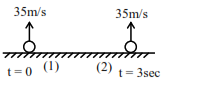# Two spherical balls having equal masses with radius ofQuestion:

Two spherical balls having equal masses with radius of $5 \mathrm{~cm}$ each are thrown upwards along the same vertical direction at an interval of $3 \mathrm{~s}$ with the same initial velocity of $35 \mathrm{~m} / \mathrm{s}$, then these balls collide at a height of $\mathrm{m} .\left(\right.$ Take $\left.\mathrm{g}=10 \mathrm{~m} / \mathrm{s}^{2}\right)$

Solution:When both balls will collied

$\mathrm{y}_{1}=\mathrm{y}_{2}$

$35 \mathrm{t}-\frac{1}{2} \times 10 \times \mathrm{t}^{2}=35(\mathrm{t}-3)-\frac{1}{2} \times 10 \times(\mathrm{t}-3)^{2}$

$35 \mathrm{t}-\frac{1}{2} \times 10 \times \mathrm{t}^{2}=35 \mathrm{t}-105-\frac{1}{2} \times 10 \times \mathrm{t}^{2}$

$-\frac{1}{2} \times 10 \times 3^{2}+\frac{1}{2} \times 10 \times 6 \mathrm{t}$

$0=150-30 t$

$t=5 \mathrm{sec}$

$\therefore$ Height at which both balls will collied

$h=35 t-\frac{1}{2} \times 10 \times t^{2}$

$=35 \times 5-\frac{1}{2} \times 10 \times 5^{2}$

$h=50 \mathrm{~m}$

Ans. $50.00$